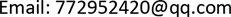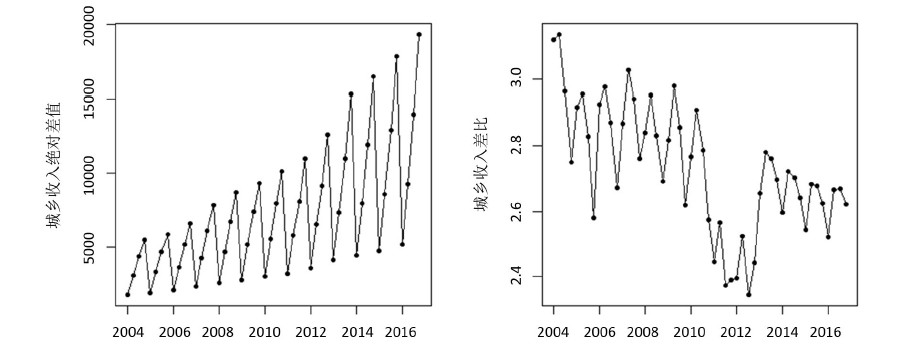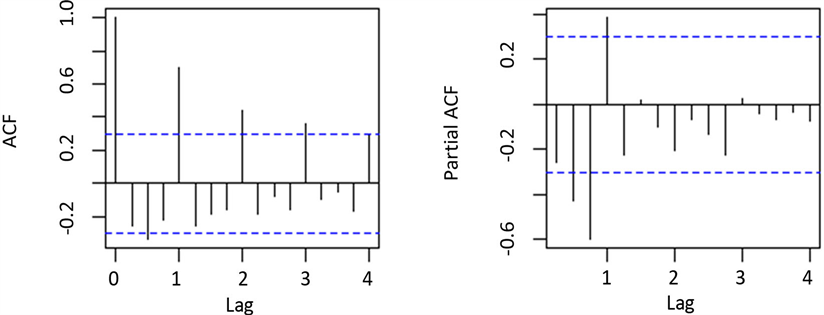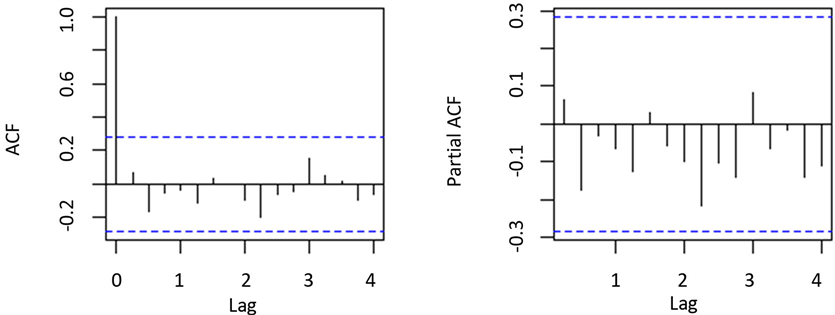﻿ 湖南省城乡居民收入差距预测—基于ARIMA-MA模型 Forecast of Income Gap between Urban and Rural Residents in Hunan Province—A Study Based on ARIMA-GM Combination Model

Statistics and Application
Vol.07 No.03(2018), Article ID:25597,10 pages
10.12677/SA.2018.73039

Forecast of Income Gap between Urban and Rural Residents in Hunan Province

—A Study Based on ARIMA-GM Combination Model

Wenxin Qu, Xiangdong Liu

Jinan University, Guangzhou GuangdongReceived: Jun. 3rd, 2018; accepted: Jun. 20th, 2018; published: Jun. 27th, 2018ABSTRACT

The income gap between urban and rural areas has always been a key issue in the five-year plan. In this article, by collecting the quarterly data of urban and rural residents’ income in Hunan Province from 2004 to 2017, we establish and compare the seasonal multiplicative ARIMA model, GM(1,1) model, and ARIMA-GM combined model, finally the ARIMA-GM combination model is applied to analysis and forecast the urban-rural income gap in Hunan from 2018 to 2019. The result shows that the ARIMA-GM combination model can solve the problem of estimating and predicting the income gap in Hunan province, and the prediction accuracy is high, so as to provide an important basis for the government to formulate relevant policies.

Keywords:Urban-Rural Income Gap, Multiplicative Seasonal ARIMA Model, GM(1,1) Model, ARIMA-GM(1,1) Combination Model, Forecast

—基于ARIMA-MA模型Copyright © 2018 by authors and Hans Publishers Inc.1. 引言

“十三五规划”中，三农问题、收入分配仍然是亟待解决的热点问题。农民问题一直是制约生产力发展的一个重要因素，城乡居民收入差距是衡量我们经济社会发展的重要指标。分析和预测城乡居民收入差距的动态变化过程，一方面可以了解一个地区经济社会的发展平衡性，另一方面可以为国家科学制定发展农村经济的政策，缩小城乡差距，促进经济增长提供参考意见，有利于实现城镇化以及全面小康社会这一目标。

2. 理论与方法

2.1. 季节乘法ARIMA模型

ARIMA模型，即求和–自回归–移动平均模型，简称B-J模型。在ARIMA模型中，变量的未来取值可以表达为过去若干取值和随机误差的线性函数，该模型自产生以来，已广泛应用于各领域。如时间序列在进行D阶季节差分前进行了d阶逐期差分后达到平稳，则可以建立随机季节模型与ARIMA模型的结合-ARIMA乘法季节模型。

$\left\{\begin{array}{l}\varphi \left(B\right)=1-{\varphi }_{1}B-{\varphi }_{2}{B}^{2}-\cdots -{\varphi }_{p}{B}^{p}\hfill \\ \Phi \left(B\right)=1-{\Phi }_{1}{B}^{{}_{S}}-{\Phi }_{2}{B}^{{2}_{S}}-\cdots -{\Phi }_{P}{B}^{{P}_{S}}\hfill \\ \psi \left(B\right)=1-{\psi }_{1}B-{\psi }_{2}{B}^{2}-\cdots -{\psi }_{q}{B}^{q}\hfill \\ \Theta \left(B\right)=1-{\Theta }_{1}{B}^{{}_{S}}-{\Theta }_{2}{B}^{{2}_{S}}-\cdots -{\Theta }_{P}{B}^{{Q}_{S}}\hfill \end{array}$

${\nabla }_{s}{Y}_{t}={Y}_{t}-{Y}_{t-s}$ (1)

${W}_{t}={\nabla }^{d}{\nabla }_{s}^{D}{Y}_{t}$ (2)

2.2. 分组GM(1,1)模型

$B=\left[\begin{array}{cc}-1/2\left({X}_{i}^{\left(1\right)}\left(1\right)+{X}_{i}^{\left(1\right)}\left(2\right)\right)& 1\\ -1/2\left({X}_{i}^{\left(1\right)}\left(2\right)+{X}_{i}^{\left(1\right)}\left(3\right)\right)& 1\\ ⋮& ⋮\\ -1/2\left({X}_{i}^{\left(1\right)}\left(k-1\right)+{X}_{i}^{\left(1\right)}\left(k\right)\right)& 1\end{array}\right]$${y}_{N}=\left[\begin{array}{c}{x}_{i}^{\left(0\right)}\left(2\right)\\ {x}_{i}^{\left(0\right)}\left(3\right)\\ ⋮\\ {X}_{i}^{\left(0\right)}\left(k\right)\end{array}\right]$

${\stackrel{^}{X}}_{i}^{\left(0\right)}\left(k\right)={\stackrel{^}{X}}_{i}^{\left(1\right)}\left(k\right)-{\stackrel{^}{X}}_{i}^{\left(1\right)}\left(k-1\right),\text{\hspace{0.17em}}\left(k=1,2,3,\cdots ,n\right)$ (3)

2.3. ARIMA-GM组合模型

$\left\{\begin{array}{l}{X}_{0t}=\underset{i=1}{\overset{n}{\sum }}{w}_{i}{\phi }_{i}\\ \underset{i=1}{\overset{n}{\sum }}{w}_{i}=1\end{array}$ (4)

${\lambda }_{i}=\frac{{a}_{i}{w}_{i}}{\underset{i-1}{\overset{J}{\sum }}{a}_{i}{w}_{i}},$ (5)

${X}_{0t}={\lambda }_{1}{\phi }_{1}+{\lambda }_{2}{\phi }_{2}+\cdots +{\lambda }_{J}{\phi }_{J}$ (6)

3. 实证分析

3.1. 数据的来源与处理Table 2. Data of income gap between urban and rural areas in Hunan ProvinceFigure 1. Curve: income gap between urban and rural areas in Hunan Province

3.2. 乘法季节ARMIA模型的预测

3.2.1. 时间序列平稳化

3.2.2. 模型定阶和检验

$\nabla {\nabla }^{4}{y}_{1t}=\frac{1+B}{\left(1-0.5323B\right)\left(1-0.6974{B}^{4}\right)}{\epsilon }_{t}$ (7)

6，12的情况下，P值为0.8472和0.8286，均大于显著水平0.05，因此不能拒绝残差不存在自相关性的零假设。图3为拟合模型(1)残差的自相关系数和偏自相关系数图。图形显示，时间序列模型残差的

Ljung-Box统计量在滞后各期都没有显著的自相关，残差为白噪声过程。因此建立 $\text{ARIMA}\left(1,1,1\right)×{\left(1,1,0\right)}_{4}$

3.3. GM(1,1)模型的预测Figure 2. Two absolute difference autocorrelation diagrams and partial autocorrelation diagrams for differential incomeFigure 3. Autocorrelation diagrams and partial autocorrelation diagrams of model (7)

${\stackrel{^}{X}}_{i}^{\left(1\right)}\left(k+1\right)=19304.8475{\text{e}}^{0.0933k}+17549.1475$ (8)

$k=1,2,3,\cdots ,n$ ，通过 ${\stackrel{^}{X}}_{i}^{\left(0\right)}\left(k\right)={\stackrel{^}{X}}_{i}^{\left(1\right)}\left(k\right)-{\stackrel{^}{X}}_{i}^{\left(1\right)}\left(k-1\right),\left(k=1,2,3,\cdots ,n\right)$ 即可得出第一季度城乡收入差距预测值。重复上述过程，分别求出第二季度、第三季度和第四季度预测值。

3.4. ARIMA-GM组合模型的预测Table 5. Model evaluation results of income difference ratioTable 6. Forecast of urban-rural income disparity in Hunan province from 2018 to 2019

${\lambda }_{2}=0.0814733$ 。同样用平均相对误差对三组模型进行模型评估，评估结果如表5所示。组合模型的拟合效果始终优于单个模型的拟合效果，可用于短期预测。

4. 结论

Forecast of Income Gap between Urban and Rural Residents in Hunan Province—A Study Based on ARIMA-GM Combination Model[J]. 统计学与应用, 2018, 07(03): 330-339. https://doi.org/10.12677/SA.2018.73039

1. 1. 傅德红, 等. 我国城乡人均收入差距的实证分析和预测[J]. 社会视野, 2008(8).

2. 2. Tu, X.L. (2011) Comparative Research on Prediction Model of China’s Urban-Rural Residents’ Income Gap. Asian Agricultural Research, 3, 88-91.

3. 3. 辜子寅. 基于ARMA-GM组合模型的江苏省城乡收入差距预测[J]. 常熟理工学院学报, 2012, 26(8): 18-21.

4. 4. 龚承刚, 王梦, 谢航. 基于ARIMA模型的湖北省城乡居民收入差距的预测[J]. 统计与决策, 2014(15): 94-97.

5. 5. 汪潘义, 吴凤平. 灰色预测模型在季节波动预测中的应用[J]. 统计与决策, 2009(24): 22-23.

6. 6. 李佩, 彭斯俊. 一种新的组合权重在组合预测模型中的应用[J]. 河南科技大学学报, 2018, 39(2): 87-98.